# ML Aggarwal Class 10 Solutions for ICSE Maths Chapter 5 Quadratic Equations in One Variable Ex 5.4

## ML Aggarwal Class 10 Solutions for ICSE Maths Chapter 5 Quadratic Equations in One Variable Ex 5.4

ML Aggarwal Class 10 Solutions for ICSE Maths Chapter 5 Quadratic Equations in One Variable Ex 5.4

Question 1.
Find the discriminant of the following equations and hence find the nature of roots:
(i) 3x² – 5x – 2 = 0
(ii) 2x² – 3x + 5 = 0
(iii) 7x² + 8x + 2 = 0
(iv) 3x² + 2x – 1 = 0
(v) 16x² – 40x + 25 = 0
(vi) 2x² + 15x + 30 = 0.
Solution: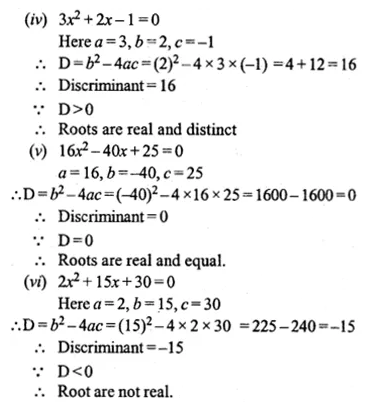Question 2.
Discuss the nature of the roots of the following quadratic equations:
(i) x² – 4x – 1 = 0
(ii) 3x² – 2x + $$\\ \frac { 1 }{ 3 }$$ = 0
(iii) 3x² – 4√3x + 4 = 0
(iv) x² – $$\\ \frac { 1 }{ 2 } x$$ + 4 = 0
(v) – 2x² + x + 1 = 0
(vi) 2√3x² – 5x + √3 = 0
Solution:Question 3.
Find the nature of the roots of the following quadratic equations:
(i) x² – $$\\ \frac { 1 }{ 2 } x$$ – $$\\ \frac { 1 }{ 2 }$$ = 0
(ii) x² – 2√3x – 1 = 0 If real roots exist, find them.
Solution:Question 4.
Without solving the following quadratic equation, find the value of ‘p’ for which the given equations have real and equal roots:
(i) px² – 4x + 3 = 0
(ii) x² + (p – 2)x + p = 0.
Solution:Question 5.
Find the value (s) of k for which each of the following quadratic equation has equal roots:
(i) kx² – 4x – 5 = 0
(ii) (k – 4) x² + 2(k – 4) x + 4 = 0
Solution: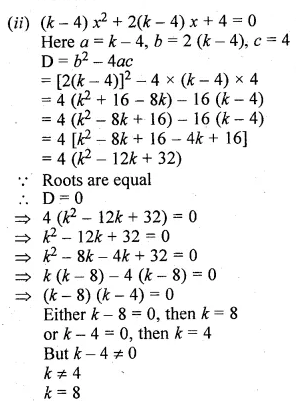Question 6.
Find the value(s) of m for which each of the following quadratic equation has real and equal roots:
(i) (3m + 1)x² + 2(m + 1)x + m = 0
(ii) x² + 2(m – 1) x + (m + 5) = 0
Solution:Question 7.
Find the values of k for which each of the following quadratic equation has equal roots:
(i) 9x² + kx + 1 = 0
(ii) x² – 2kx + 7k – 12 = 0
Also, find the roots for those values of k in each case.
Solution: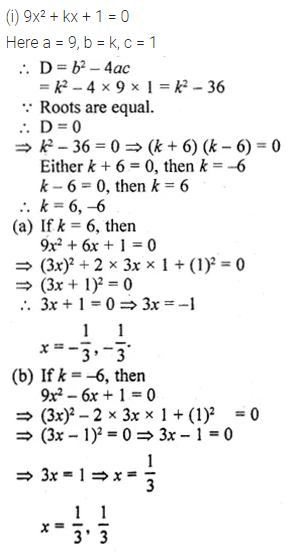Question 8.
Find the value(s) of p for which the quadratic equation (2p + 1)x² – (7p + 2)x + (7p – 3) = 0 has equal roots. Also find these roots.
Solution: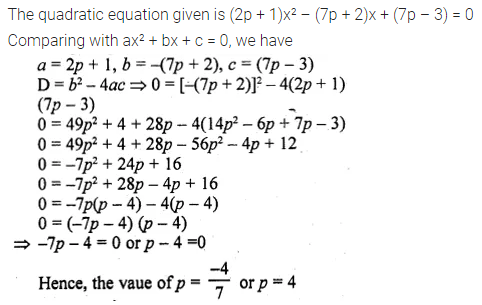Question 9.
If – 5 is a root of the quadratic equation 2x² + px – 15 = 0 and the quadratic equation p(x² + x) + k = 0 has equal roots, find the value of k.
Solution:Question 10.
Find the value(s) of p for which the equation 2x² + 3x + p = 0 has real roots.
Solution:Question 11.
Find the least positive value of k for which the equation x² + kx + 4 = 0 has real roots.
Solution: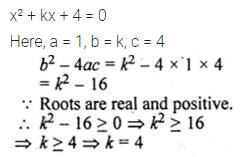Question 12.
Find the values of p for which the equation 3x² – px + 5 = 0 has real roots.
Solution: